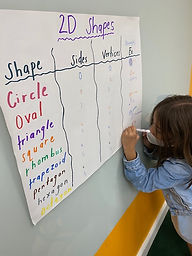## Ms. Dawn

### Target 1​

###### Lesson Type:

Continuation

Geometry

:

Shape

Measure, draw, and classify angles.

###### 1:

Accurately measure angles.

###### 2:

Identify angles as acute, obtuse, or right.

###### 3:

Understand that angles have types based on their degree measures (acute, obtuse, and right).

4th

###### Vocabulary:

Acute angle, Obtuse angle, Right angle, Straight angle, Reflex angle, Degrees

Activities:

1. Reviewed/discussed with students the definition of an acute angle, obtuse angle, right angle, straight angle and reflex angle.

2. Students walked around the table using a protractor to measure a variety of angles that were created by painters tape. They wrote the angle measurement and named the type of angle. Students used markers and wrote it by the corresponding angle.### Home Exploration

###### Guiding Questions:## Absent Students:

### Target 2

:

###### 1:

Identify polygons by their defining attributes.

###### 2:

Define each type of quadrilateral (square, rectangle, parallelogram, rhombus, and trapezoid).

###### 3:

Identify quadrilaterals by their defining attributes (number of sides, number of vertices, vertices angle measurements, line types, and side lengths).

###### 4:

Identify triangles by their defining attributes (number of sides, number of vertices, vertices angle measurements, and side lengths).

4th

###### Vocabulary:

2D shape, Sides, Vertices, Oval, Circle, Triangle, Rectangle, Square, Trapezoid, Rhombus, Pentagon, Hexagon, Octagon

Activities:

Discussed what defines a 2D shape. Then, students named 2D shapes. A chart was created with all the different 2D shapes. Students used sticks and Play Doh to create a variety of 2D shapes. Then, students discussed the vocabulary of side and vertices in a 2D shape. Once students understood the meaning, they filled in the chart. They stated how many sides, vertices, and lastly gave a real-life example.### Home Exploration

###### Guiding Questions:### Target 3

:

4th

###### Vocabulary:

3D shape, Faces, Edges, Vertices, Cube, Rectangular prism, Triangular prism, Square pyramid, Cylinder, Cone, Sphere, Hexagonal prism, Pentagonal prism

Activities:

1. Discussed what defines a 3D shape.

2. Observed and looked at a variety of 3D shapes.

3. Discussed math vocabulary faces, vertices, and edges.

4. Using one of the 3D foam shapes, discussed the different math terms faces, vertices and edges by students placing their finger where that term applied.

5. Students created a variety of 3D shapes with the sticks and Play Doh.

6. Students filled out a 3D shape chart where they needed to state the name of the shape, how many edges, how many vertices, and how many faces each had.### Home Exploration